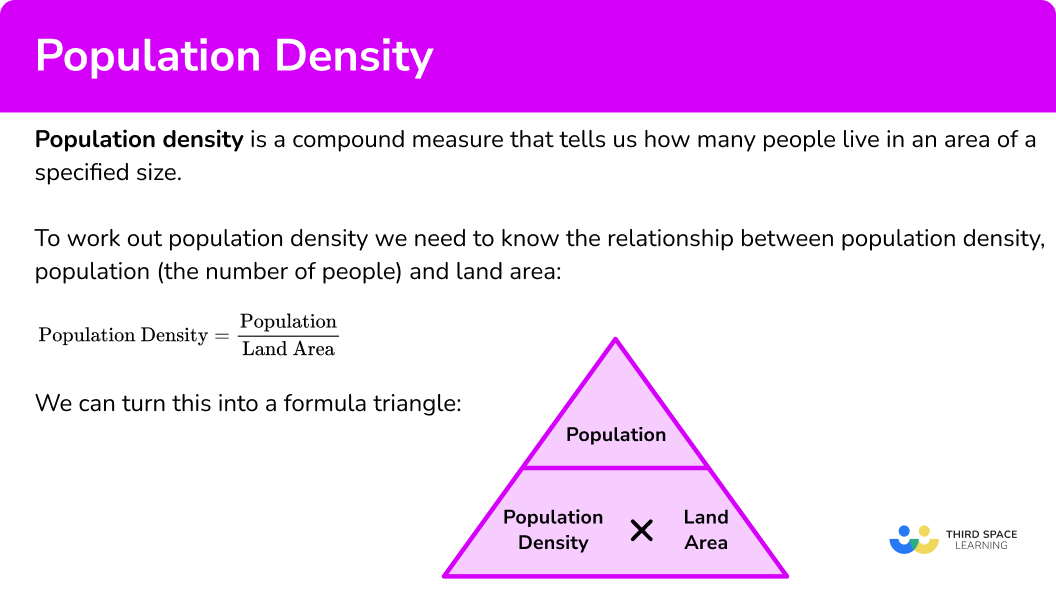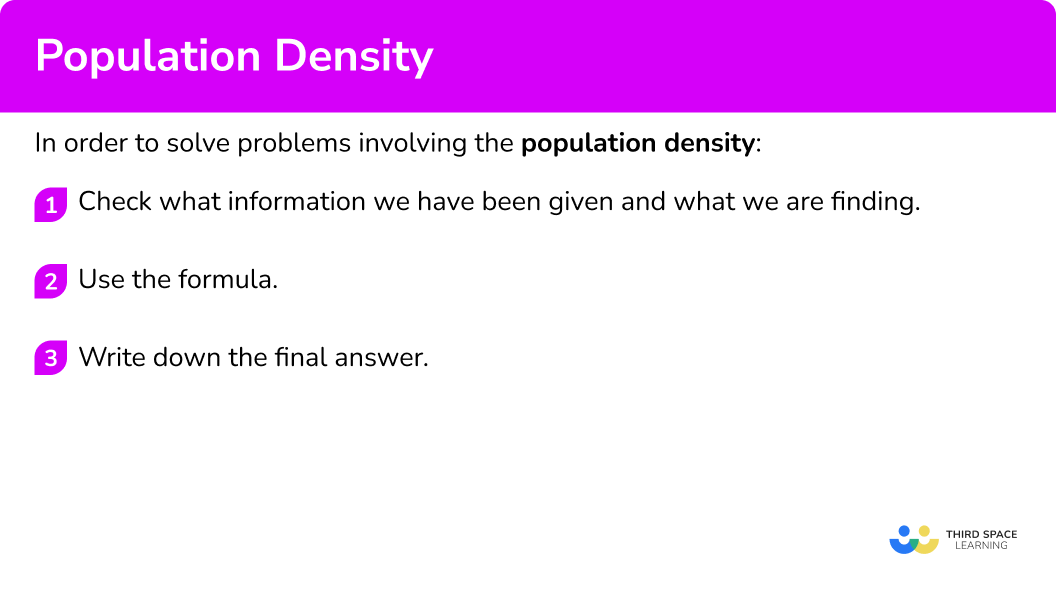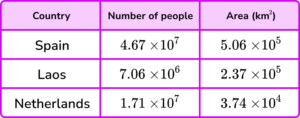# Population Density

Here we will learn about population density, including how to calculate population density and how to solve problems involving population density.

There are also population density worksheets based on Edexcel, AQA and OCR exam questions, along with further guidance on where to go next if you’re still stuck.

## What is population density?

Population density is a compound measure that tells us how many people live in an area of a specified size.

If an area has a high population density it is crowded, for example in a city.

If an area has a low population density it is sparsely populated, for example a mountainous region.

To work out population density we need to know the relationship between population density, population (the number of people) and land area.

\text{Population density}=\frac{\text{population}}{\text{land area}}

We can turn this into a formula triangle,

Population density can be used to make population estimates. The units are often people/km^2 (people per square kilometre). Occasionally the units might be people per square mile.

You may study more about world population, population growth, urbanisation and demographics in geography.

### What is population density?## How to use population density

In order to solve problems involving population density:

1. Check what information we have been given and what we are finding.
2. Use the formula.
3. Write down the final answer.

### Explain how to use population density### Related lessons oncompound measures

Population density is part of our series of lessons to support revision on compound measures. You may find it helpful to start with the main compound measures lesson for a summary of what to expect, or use the step by step guides below for further detail on individual topics. Other lessons in this series include:

## Population density examples

### Example 1: calculating population density

Malta has a population of 440 \ 000 and an area of 316 \ km^{2}. Calculate the population density of Malta. Give your answer to the nearest integer.

1. Check what information we have been given and what we are finding.

We have been given the population and the land area. We need to find the population density.

2Use the formula.

The formula is

\text{Population density}=\frac{\text{population}}{\text{land area}} .

We can substitute in the values and work out the population density.

\text{Population density}=\frac{\text{population}}{\text{land area}}=\frac{440 \ 000}{316}=1392.405…

The population density of Malta is \bf{1392} people \ per \ km^{2} (to the nearest integer).

### Example 2: calculating population density

Australia has a population of 24 \ 900 \ 000 and an area of 7 \ 690 \ 000 \ km^{2}. Calculate the population density of Australia. Give your answer to 3 significant figures.

We have been given the population and the land area. We need to find the population density.

The formula is

\text{Population density}=\frac{\text{population}}{\text{land area}} .

We can substitute in the values and work out the population density.

\text{Population density}=\frac{\text{population}}{\text{land area}}=\frac{24\ 900 \ 000}{7 \ 690 \ 000}=3.2379…

The population density of Australia is \bf{3.24} people \ per \ km^2 (to 3 significant figures).

### Example 3: calculating population

Hong Kong has a population density of 6700 \ people/km^2 and an area of 1100 \ km^{2}. Calculate the population of Hong Kong.

We have been given the population density and the land area. We need to find the population.

The formula is

\text{Population density}=\frac{\text{population}}{\text{land area}} .

We can substitute in the values and work out the population density.

\begin{aligned} \text{Population density}&=\frac{\text{population}}{\text{land area}}\\\\ 6 \ 700 &=\frac{\text{population}}{1 \ 100}\\\\ \text{Population}&=6 \ 700 \times 1 \ 100\\\\ \text{Population}&=7 \ 370 \ 000 \end{aligned}

The population of Hong Kong is 7 \ 370 \ 000 people.

### Example 4: calculating population

Rwanda has a population density of 470 \ people/km^2 and an area of 26 \ 000 \ km^{2}. Calculate the population of Rwanda.

We have been given the population density and the land area. We need to find the population.

The formula is

\text{Population density}=\frac{\text{population}}{\text{land area}} .

We can substitute in the values and work out the population density.

\begin{aligned} \text{Population density}&=\frac{\text{population}}{\text{land area}}\\\\ 470 &=\frac{\text{population}}{26 \ 000}\\\\ \text{Population}&=470 \times 26 \ 000 \\\\ \text{Population}&=12 \ 220 \ 000 \end{aligned}

The population of Rwanda is \bf{12 \ 220 \ 000} people.

### Example 5: calculating land area

New York has a population density of 11 \ 300 \ people/km^2 and a population of 8.8 million people. Calculate the land area of New York. Give your answer to 3 significant figures.

We have been given the population density and the population. We need to find the land area

The formula is

\text{Population density}=\frac{\text{population}}{\text{land area}} .

We can substitute in the values and work out the population density.

\begin{aligned} \text{Population density}&=\frac{\text{population}}{\text{land area}}\\\\ 11 \ 300 &=\frac{8 \ 800 \ 000}{\text{land area}}\\\\ \text{Land area}&=8 \ 800 \ 000\div 11 \ 300\\\\ \text{Land area}&=778.76… \end{aligned}

The land area of New York is \bf{779} km^2 (to 3 significant figures).

### Example 6: calculating land area

Pakistan has a population density of 267 \ people/km^2 and a population of 2.12 \times 10^8 people. Calculate the land area of Pakistan. Give your answer in standard form to 3 significant figures.

We have been given the population density and the population. We need to find the land area.

The formula is

\text{Population density}=\frac{\text{population}}{\text{land area}} .

We can substitute in the values and work out the population density.

\begin{aligned} \text{Population density}&=\frac{\text{population}}{\text{land area}}\\\\ 267 &=\frac{2.12\times 10^8}{\text{land area}}\\\\ \text{Land area}&=2.12\times 10^8\div 267\\\\ \text{Land area}&=794 \ 007.49… \end{aligned}

\text{Land area}=794\ 007.49…=794\ 000=7.94\times 10^5

The land area of Pakistan is 7.94 \times 10^5 \ km^2 (to 3 significant figures).

### Common misconceptions

• Make sure you use the formula wisely

Make sure you divide or multiply appropriately. When dividing, make sure the population is the dividend (the number being divided).

• Check the units

The units usually involve km^{2}, but another square unit may be used such as miles^2 or m^{2}.

• Check what form the final answer needs to be in

### Practice population density questions

1. Calculate the population density of Nepal given that the population of Nepal is 28 \ 100 \ 000 and the land area is 147 \ 000 \ km^{2}. Give your answer to 3 significant figures.

193 \ people/km^2191 \ people/km^2192 \ people/km^2194 \ people/km^2Population density =\frac{\text{population}}{\text{land area}}=\frac{28 \ 100 \ 00}{147 \ 000}=191.156…

The answer needs rounding to 3 significant figures.

191.156…=191 to 3 significant figures.

So the population density of Nepal is 191 \ people/km^2 .

2. Calculate the population density of Monaco given that the population of Monaco is 3.87 \times 10^4 and the land area is 2 \ km^{2}. Give your answer as an ordinary number to 3 significant figures.

19 \ 300 \ people/km^21 \ 940 \ people/km^219 \ 400 \ people/km^2194 \ people/km^2\text{Population density}=\frac{\text{population}}{\text{land area}}=\frac{3.87\times 10^4}{2}=19 \ 350 .

The answer needs rounding to 3 significant figures.

19 \ 350=19 \ 400 to 3 significant figures.

The population density of Monaco is 19 \ 400 \ people/km^{2}.

3. Calculate the population of Nigeria given that the population density of Nigeria is 212 \ people/km^2 and the land area is 924 \ 000 \ km^{2}. Give your answer to the nearest million.

4 million is the number of people who live in Nigeria.40 million is the number of people who live in Nigeria.2 million is the number of people who live in Nigeria.20 million is the number of people who live in Nigeria.To find the population, multiply the population density by the land area.

\text{Population}=\text{population density}\times \text{land area}= 212\times 924 \ 000=19 \ 588 \ 000 .

The answer needs giving to the nearest million.

19 \ 588 \ 000 is 20 million to the nearest million.

4. Calculate the population of Venezuela given that the population density is 32 \ people/km^2 and the land area of Venezuela is 9.12 \times 10^5 \ km^{2}. Give your answer in standard form correct to 3 significant figures.

2.91 \times 10^6 is the number of people living in Venezuela.2.91 \times 10^7 is the number of people living in Venezuela.2.92 \times 10^7 is the number of people living in Venezuela.2.92 \times 10^6 is the number of people living in Venezuela.To find the population, multiply the population density by the land area.

\text{Population}=\text{population density}\times \text{land area}=32\times (9.12\times 10^5)=29 \ 184 \ 000 .

Then we need to write the final answer in standard form and round to 3 significant figures.

29 \ 184 \ 000= 2.9184\times 10^7=2.92\times 10^7 to 3 significant figures.
So, the population of Venezuela is 2.92\times 10^7.

5. Calculate the land area of Taiwan when the population density is 652 \ people/km^{2} and the population of Taiwan is 23.6 million. Give your answer to 3 significant figures.

36 \ 200 \ km^2 is the land area of Taiwan.36 \ 200 \ miles^2 is the land area of Taiwan.3 \ 620 \ miles^2 is the land area of Taiwan.3 \ 620 \ km^2 is the land area of Taiwan.To find the land area, divide the population by the population density.

\text{Land area}=\frac{\text{population}}{\text{population density}}=\frac{23 \ 600 \ 000}{652}=36 \ 196.3… .

Then we need to write the final answer rounded to 3 significant figures.

36 \ 196.3…=36 \ 200 to 3 significant figures.
The units for the land area will be km^2 since km^2 was part of the units for the population density.

6. Calculate the land area when the population density of Indonesia is 140 \ people/km^2 and the population of Indonesia 2.68 \times 10^{8}. Give your answer in standard form correct to 3 significant figures.

1.92\times 10^5 \ km^2 is the land area of Indonesia.1.92\times 10^6 \ km^2 is the land area of Indonesia.1.91\times 10^6 \ km^2 is the land area of Indonesia.1.91\times 10^5 \ km^2 is the land area of Indonesia.To find the land area, divide the population by the population density.

\text{Land area}=\frac{\text{population}}{\text{population density}}=\frac{2.68\times 10^8}{140}=1 \ 914 \ 285.7…

Then we need to write the final answer in standard form and round to 3 significant figures.

1 \ 914 \ 285.7… = 1.9142857…\times 10^6=1.91\times 10^6 to 3 significant figures.

### Population density GCSE questions

1. Bangladesh has a population of 161 \ 000 \ 000 people. The population density is 1000 people per km^2. Calculate the area of Bangladesh.

(2 marks)

161 \ 000 \ 000 \div 1000

(1)

161 \ 000 \ km^2

(1)

2. Here is some information about the populations and areas of 3 different towns.Which country has the highest population density? You must show your workings.

(2 marks)

\begin{aligned} &\text{Spain:}\ 4.67\times 10^7 \div 5.06\times 10^5=92.292… \\\\ &\text{Laos:}\ 7.06\times 10^6 \div 2.37\times 10^5=29.789… \\\\ &\text{Netherlands:}\ 1.71\times 10^7 \div 3.74\times 10^4=457.219… \end{aligned}

(1)

Netherlands has the highest population density.

(1)

3. (a) A village has an area of 5 \ km^2 and a population density of 600 \ people \ per \ km^{2}.

Calculate the number of people living in the village.

(b) A new estate is built. It has a population density of 800 \ people \ per \ km^{2}. \ 500 people live in the new estate.

Calculate the overall population density of the village and the new estate.

(5 marks)

(a)

5 \times 600

(1)

3000 people

(1)

(b)

500 \div 800=0.625

(1)

\frac{3000+500}{5+0.625}

(1)

=622.222… = 622 \ people \ per \ km^2

(1)

## Learning checklist

You have now learned how to:

• Calculate the population density
• Solve problems involving population density

## Still stuck?

Prepare your KS4 students for maths GCSEs success with Third Space Learning. Weekly online one to one GCSE maths revision lessons delivered by expert maths tutors.

Find out more about our GCSE maths tuition programme.